In this tutorial you will learn how to use OpenCV to detect text in natural scene images using the EAST text detector.

OpenCV’s EAST text detector is a deep learning model, based on a novel architecture and training pattern. It is capable of (1) running at near real-time at 13 FPS on 720p images and (2) obtains state-of-the-art text detection accuracy.

In the remainder of this tutorial you will learn how to use OpenCV’s EAST detector to automatically detect text in both images and video streams.

To discover how to apply text detection with OpenCV, just keep reading!

Looking for the source code to this post?

## OpenCV Text Detection (EAST text detector)

In this tutorial, you will learn how to use OpenCV to detect text in images using the EAST text detector.

The EAST text detector requires that we are running OpenCV 3.4.2 or OpenCV 4 on our systems — if you do not already have OpenCV 3.4.2 or better installed, please refer to my OpenCV install guides and follow the one for your respective operating system.

In the first part of today’s tutorial, I’ll discuss why detecting text in natural scene images can be so challenging.

From there I’ll briefly discuss the EAST text detector, why we use it, and what makes the algorithm so novel — I’ll also include links to the original paper so you can read up on the details if you are so inclined.

Finally, I’ll provide my Python + OpenCV text detection implementation so you can start applying text detection in your own applications.

### Why is natural scene text detection so challenging?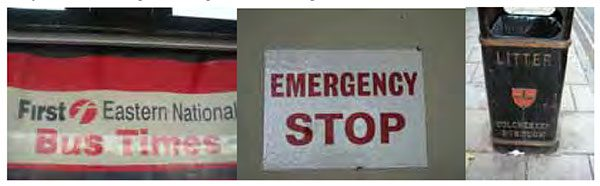Figure 1: Examples of natural scene images where text detection is challenging due to lighting conditions, image quality, and non-planar objects (Figure 1 of Mancas-Thillou and Gosselin).

Detecting text in constrained, controlled environments can typically be accomplished by using heuristic-based approaches, such as exploiting gradient information or the fact that text is typically grouped into paragraphs and characters appear on a straight line. An example of such a heuristic-based text detector can be seen in my previous blog post on Detecting machine-readable zones in passport images.

Natural scene text detection is different though — and much more challenging.

Due to the proliferation of cheap digital cameras, and not to mention the fact that nearly every smartphone now has a camera, we need to be highly concerned with the conditions the image was captured under — and furthermore, what assumptions we can and cannot make. I’ve included a summarized version of the natural scene text detection challenges described by Celine Mancas-Thillou and Bernard Gosselin in their excellent 2017

paper, Natural Scene Text Understanding below:

• Image/sensor noise: Sensor noise from a handheld camera is typically higher than that of a traditional scanner. Additionally, low-priced cameras will typically interpolate the pixels of raw sensors to produce real colors.
• Viewing angles: Natural scene text can naturally have viewing angles that are not parallel to the text, making the text harder to recognize.
• Blurring: Uncontrolled environments tend to have blur, especially if the end user is utilizing a smartphone that does not have some form of stabilization.
• Lighting conditions: We cannot make any assumptions regarding our lighting conditions in natural scene images. It may be near dark, the flash on the camera may be on, or the sun may be shining brightly, saturating the entire image.
• Resolution: Not all cameras are created equal — we may be dealing with cameras with sub-par resolution.
• Non-paper objects: Most, but not all, paper is not reflective (at least in context of paper you are trying to scan). Text in natural scenes may be reflective, including logos, signs, etc.
• Non-planar objects: Consider what happens when you wrap text around a bottle — the text on the surface becomes distorted and deformed. While humans may still be able to easily “detect” and read the text, our algorithms will struggle. We need to be able to handle such use cases.
• Unknown layout: We cannot use any a priori information to give our algorithms “clues” as to where the text resides.

As we’ll learn, OpenCV’s text detector implementation of EAST is quite robust, capable of localizing text even when it’s blurred, reflective, or partially obscured: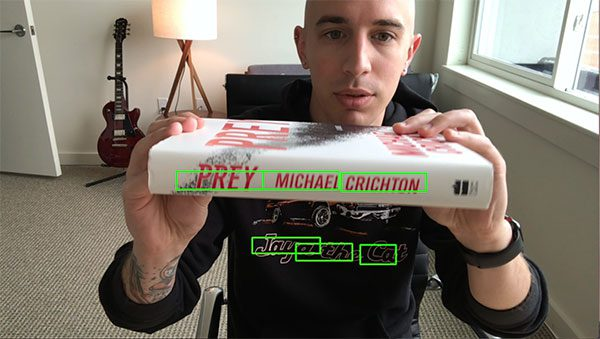Figure 2: OpenCV’s EAST scene text detector will detect even in blurry and obscured images.

I would suggest reading Mancas-Thillou and Gosselin’s work if you are further interested in the challenges associated with text detection in natural scene images.

### The EAST deep learning text detector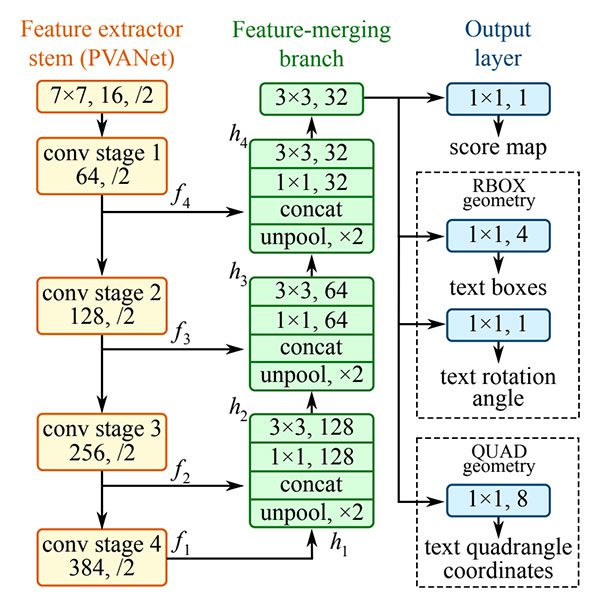Figure 3: The structure of the EAST text detection Fully-Convolutional Network (Figure 3 of Zhou et al.).

With the release of OpenCV 3.4.2 and OpenCV 4, we can now use a deep learning-based text detector called EAST, which is based on Zhou et al.’s 2017

We call the algorithm “EAST” because it’s an: Efficient and Accurate Scene Text detection pipeline.

The EAST pipeline is capable of predicting words and lines of text at arbitrary orientations on 720p images, and furthermore, can run at 13 FPS, according to the authors.

Perhaps most importantly, since the deep learning model is end-to-end, it is possible to sidestep computationally expensive sub-algorithms that other text detectors typically apply, including candidate aggregation and word partitioning.

To build and train such a deep learning model, the EAST method utilizes novel, carefully designed loss functions.

For more details on EAST, including architecture design and training methods, be sure to refer to the publication by the authors.

### Project structure

To start, be sure to grab the source code + images to today’s post by visiting the “Downloads” section. From there, simply use the

`tree`
terminal command to view the project structure:
```\$ tree --dirsfirst
.
├── images
│   ├── car_wash.png
│   ├── lebron_james.jpg
│   └── sign.jpg
├── frozen_east_text_detection.pb
├── text_detection.py
└── text_detection_video.py

1 directory, 6 files```

Notice that I’ve provided three sample pictures in the

`images/`
directory. You may wish to add your own images collected with your smartphone or ones you find online.

We’ll be reviewing two

`.py`
files today:
• `text_detection.py`
: Detects text in static images.
• `text_detection_video.py`
: Detects text via your webcam or input video files.

Both scripts make use of the serialized EAST model (

`frozen_east_text_detection.pb`

### Implementation notes

The text detection implementation I am including today is based on OpenCV’s official C++ example; however, I must admit that I had a bit of trouble when converting it to Python.

To start, there are no

`Point2f`
and
`RotatedRect`
functions in Python, and because of this, I could not 100% mimic the C++ implementation. The C++ implementation can produce rotated bounding boxes, but unfortunately the one I am sharing with you today cannot.

Secondly, the

`NMSBoxes`
function does not return any values for the Python bindings (at least for my OpenCV 4 pre-release install), ultimately resulting in OpenCV throwing an error. The
`NMSBoxes`
function may work in OpenCV 3.4.2 but I wasn’t able to exhaustively test it.

I got around this issue my using my own non-maxima suppression implementation in imutils, but again, I don’t believe these two are 100% interchangeable as it appears

`NMSBoxes`

Given all that, I’ve tried my best to provide you with the best OpenCV text detection implementation I could, using the working functions and resources I had. If you have any improvements to the method please do feel free to share them in the comments below.

### Implementing our text detector with OpenCV

Before we get started, I want to point out that you will need at least OpenCV 3.4.2 (or OpenCV 4) installed on your system to utilize OpenCV’s EAST text detector, so if you haven’t already installed OpenCV 3.4.2 or better on your system, please refer to my OpenCV install guides.

Next, make sure you have

`imutils`
`\$ pip install --upgrade imutils`

At this point your system is now configured, so open up

`text_detection.py`
and insert the following code:
```# import the necessary packages
from imutils.object_detection import non_max_suppression
import numpy as np
import argparse
import time
import cv2

# construct the argument parser and parse the arguments
ap = argparse.ArgumentParser()
help="path to input image")
help="path to input EAST text detector")
help="minimum probability required to inspect a region")
help="resized image width (should be multiple of 32)")
help="resized image height (should be multiple of 32)")
args = vars(ap.parse_args())```

To begin, we import our required packages and modules on Lines 2-6. Notably we import NumPy, OpenCV, and my implementation of

`non_max_suppression`
from
`imutils.object_detection`
.

We then proceed to parse five command line arguments on Lines 9-20:

• `--image`
: The path to our input image.
• `--east`
: The EAST scene text detector model file path.
• `--min-confidence`
: Probability threshold to determine text. Optional with
`default=0.5`
.
• `--width`
: Resized image width — must be multiple of 32. Optional with
`default=320`
.
• `--height`
: Resized image height — must be multiple of 32. Optional with
`default=320`
.

Important: The EAST text requires that your input image dimensions be multiples of 32, so if you choose to adjust your

`--width`
and
`--height`
values, make sure they are multiples of 32!

From there, let’s load our image and resize it:

```# load the input image and grab the image dimensions
orig = image.copy()
(H, W) = image.shape[:2]

# set the new width and height and then determine the ratio in change
# for both the width and height
(newW, newH) = (args["width"], args["height"])
rW = W / float(newW)
rH = H / float(newH)

# resize the image and grab the new image dimensions
image = cv2.resize(image, (newW, newH))
(H, W) = image.shape[:2]```

On Lines 23 and 24, we load and copy our input image.

From there, Lines 30 and 31 determine the ratio of the original image dimensions to new image dimensions (based on the command line argument provided for

`--width`
and
`--height`
).

Then we resize the image, ignoring aspect ratio (Line 34).

In order to perform text detection using OpenCV and the EAST deep learning model, we need to extract the output feature maps of two layers:

```# define the two output layer names for the EAST detector model that
# we are interested -- the first is the output probabilities and the
# second can be used to derive the bounding box coordinates of text
layerNames = [
"feature_fusion/Conv_7/Sigmoid",
"feature_fusion/concat_3"]```

We construct a list of

`layerNames`
on Lines 40-42:
1. The first layer is our output sigmoid activation which gives us the probability of a region containing text or not.
2. The second layer is the output feature map that represents the “geometry” of the image — we’ll be able to use this geometry to derive the bounding box coordinates of the text in the input image

Let’s load the OpenCV’s EAST text detector:

```# load the pre-trained EAST text detector

# construct a blob from the image and then perform a forward pass of
# the model to obtain the two output layer sets
blob = cv2.dnn.blobFromImage(image, 1.0, (W, H),
(123.68, 116.78, 103.94), swapRB=True, crop=False)
start = time.time()
net.setInput(blob)
(scores, geometry) = net.forward(layerNames)
end = time.time()

# show timing information on text prediction
print("[INFO] text detection took {:.6f} seconds".format(end - start))```

We load the neural network into memory using

`cv2.dnn.readNet`
by passing the path to the EAST detector (contained in our command line
`args`
dictionary) as a parameter on Line 46.

Then we prepare our image by converting it to a

`blob`

To predict text we can simply set the

`blob`
as input and call
`net.forward`
(Lines 53 and 54). These lines are surrounded by grabbing timestamps so that we can
`print`
the elapsed time on Line 58.

By supplying

`layerNames`
as a parameter to
`net.forward`
, we are instructing OpenCV to return the two feature maps that we are interested in:
• The output
`geometry`
map used to derive the bounding box coordinates of text in our input images
• And similarly, the
`scores`
map, containing the probability of a given region containing text

We’ll need to loop over each of these values, one-by-one:

```# grab the number of rows and columns from the scores volume, then
# initialize our set of bounding box rectangles and corresponding
# confidence scores
(numRows, numCols) = scores.shape[2:4]
rects = []
confidences = []

# loop over the number of rows
for y in range(0, numRows):
# extract the scores (probabilities), followed by the geometrical
# data used to derive potential bounding box coordinates that
# surround text
scoresData = scores[0, 0, y]
xData0 = geometry[0, 0, y]
xData1 = geometry[0, 1, y]
xData2 = geometry[0, 2, y]
xData3 = geometry[0, 3, y]
anglesData = geometry[0, 4, y]```

We start off by grabbing the dimensions of the

`scores`
volume (Line 63) and then initializing two lists:
• `rects`
: Stores the bounding box (x, y)-coordinates for text regions
• `confidences`
: Stores the probability associated with each of the bounding boxes in
`rects`

We’ll later be applying non-maxima suppression to these regions.

Looping over the rows begins on Line 68.

Lines 72-77 extract our scores and geometry data for the current row,

`y`
.

Next, we loop over each of the column indexes for our currently selected row:

```# loop over the number of columns
for x in range(0, numCols):
# if our score does not have sufficient probability, ignore it
if scoresData[x] < args["min_confidence"]:
continue

# compute the offset factor as our resulting feature maps will
# be 4x smaller than the input image
(offsetX, offsetY) = (x * 4.0, y * 4.0)

# extract the rotation angle for the prediction and then
# compute the sin and cosine
angle = anglesData[x]
cos = np.cos(angle)
sin = np.sin(angle)

# use the geometry volume to derive the width and height of
# the bounding box
h = xData0[x] + xData2[x]
w = xData1[x] + xData3[x]

# compute both the starting and ending (x, y)-coordinates for
# the text prediction bounding box
endX = int(offsetX + (cos * xData1[x]) + (sin * xData2[x]))
endY = int(offsetY - (sin * xData1[x]) + (cos * xData2[x]))
startX = int(endX - w)
startY = int(endY - h)

# add the bounding box coordinates and probability score to
# our respective lists
rects.append((startX, startY, endX, endY))
confidences.append(scoresData[x])```

For every row, we begin looping over the columns on Line 80.

We need to filter out weak text detections by ignoring areas that do not have sufficiently high probability (Lines 82 and 83).

The EAST text detector naturally reduces volume size as the image passes through the network — our volume size is actually 4x smaller than our input image so we multiply by four to bring the coordinates back into respect of our original image.

I’ve included how you can extract the

`angle`
data on Lines 91-93; however, as I mentioned in the previous section, I wasn’t able to construct a rotated bounding box from it as is performed in the C++ implementation — if you feel like tackling the task, starting with the angle on Line 91 would be your first step.

From there, Lines 97-105 derive the bounding box coordinates for the text area.

We then update our

`rects`
and
`confidences`
lists, respectively (Lines 109 and 110).

We’re almost finished!

The final step is to apply non-maxima suppression to our bounding boxes to suppress weak overlapping bounding boxes and then display the resulting text predictions:

```# apply non-maxima suppression to suppress weak, overlapping bounding
# boxes
boxes = non_max_suppression(np.array(rects), probs=confidences)

# loop over the bounding boxes
for (startX, startY, endX, endY) in boxes:
# scale the bounding box coordinates based on the respective
# ratios
startX = int(startX * rW)
startY = int(startY * rH)
endX = int(endX * rW)
endY = int(endY * rH)

# draw the bounding box on the image
cv2.rectangle(orig, (startX, startY), (endX, endY), (0, 255, 0), 2)

# show the output image
cv2.imshow("Text Detection", orig)
cv2.waitKey(0)```

As I mentioned in the previous section, I could not use the non-maxima suppression in my OpenCV 4 install (

`cv2.dnn.NMSBoxes`
) as the Python bindings did not return a value, ultimately causing OpenCV to error out. I wasn’t fully able to test in OpenCV 3.4.2 so it may work in v3.4.2.

Instead, I have used my non-maxima suppression implementation available in the

`imutils`
package (Line 114). The results still look good; however, I wasn’t able to compare my output to the
`NMSBoxes`
function to see if they were identical.

Lines 117-126 loop over our bounding

`boxes`
, scale the coordinates back to the original image dimensions, and draw the output to our
`orig`
image. The
`orig`
image is displayed until a key is pressed (Lines 129 and 130).

As a final implementation note I would like to mention that our two nested

`for`
loops used to loop over the
`scores`
and
`geometry`
volumes on Lines 68-110 would be an excellent example of where you could leverage Cython to dramatically speed up your pipeline. I’ve demonstrated the power of Cython in Fast, optimized ‘for’ pixel loops with OpenCV and Python.

### OpenCV text detection results

Are you ready to apply text detection to images?

Start by grabbing the “Downloads” for this blog post and unzip the files.

From there, you may execute the following command in your terminal (taking note of the two command line arguments):

```\$ python text_detection.py --image images/lebron_james.jpg \
--east frozen_east_text_detection.pb
[INFO] text detection took 0.142082 seconds```

Your results should look similar to the following image: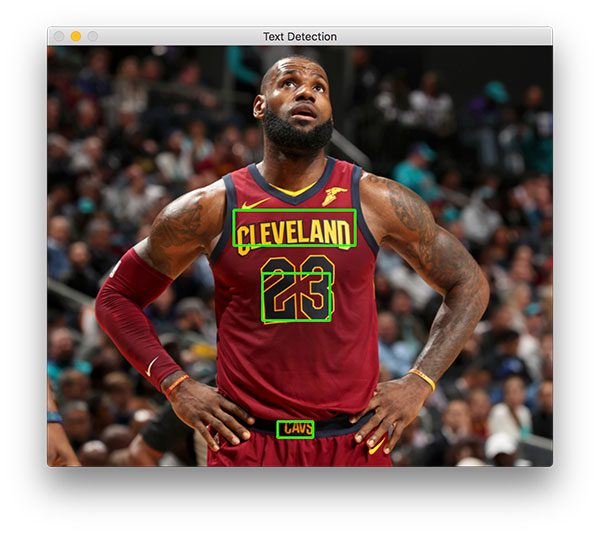Figure 4: Famous basketball player, Lebron James’ jersey text is successfully recognized with OpenCV and EAST text detection.

Three text regions are identified on Lebron James.

Now let’s try to detect text of a business sign:

```\$ python text_detection.py --image images/car_wash.png \
--east frozen_east_text_detection.pb
[INFO] text detection took 0.142295 seconds```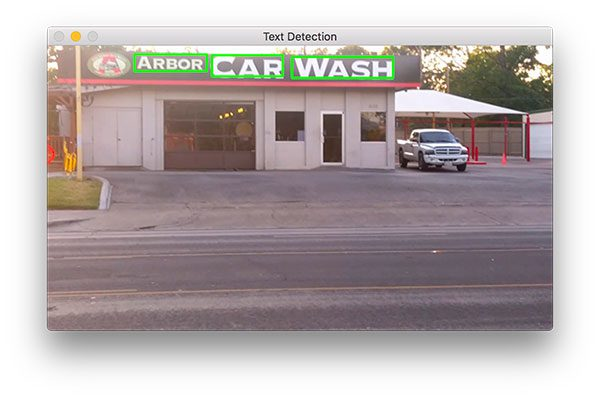Figure 5: Text is easily recognized with Python and OpenCV using EAST in this natural scene of a car wash station.

And finally, we’ll try a road sign:

```\$ python text_detection.py --image images/sign.jpg \
--east frozen_east_text_detection.pb
[INFO] text detection took 0.141675 seconds```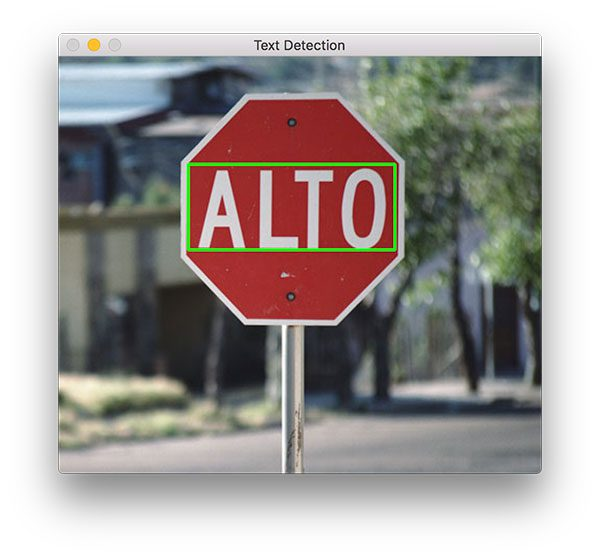Figure 6: Scene text detection with Python + OpenCV and the EAST text detector successfully detects the text on this Spanish stop sign.

This scene contains a Spanish stop sign. The word, “ALTO” is correctly detected by OpenCV and EAST.

As you can tell, EAST is quite accurate and relatively fast taking approximately 0.14 seconds on average per image.

### Text detection in video with OpenCV

Now that we’ve seen how to detect text in images, let’s move on to detecting text in video with OpenCV.

This explanation will be very brief; please refer to the previous section for details as needed.

Open up

`text_detection_video.py`
and insert the following code:
```# import the necessary packages
from imutils.video import VideoStream
from imutils.video import FPS
from imutils.object_detection import non_max_suppression
import numpy as np
import argparse
import imutils
import time
import cv2```

We begin by importing our packages. We’ll be using

`VideoStream`
to access a webcam and
`FPS`
to benchmark our frames per second for this script. Everything else is the same as in the previous section.

For convenience, let’s define a new function to decode our predictions function — it will be reused for each frame and make our loop cleaner:

```def decode_predictions(scores, geometry):
# grab the number of rows and columns from the scores volume, then
# initialize our set of bounding box rectangles and corresponding
# confidence scores
(numRows, numCols) = scores.shape[2:4]
rects = []
confidences = []

# loop over the number of rows
for y in range(0, numRows):
# extract the scores (probabilities), followed by the
# geometrical data used to derive potential bounding box
# coordinates that surround text
scoresData = scores[0, 0, y]
xData0 = geometry[0, 0, y]
xData1 = geometry[0, 1, y]
xData2 = geometry[0, 2, y]
xData3 = geometry[0, 3, y]
anglesData = geometry[0, 4, y]

# loop over the number of columns
for x in range(0, numCols):
# if our score does not have sufficient probability,
# ignore it
if scoresData[x] < args["min_confidence"]:
continue

# compute the offset factor as our resulting feature
# maps will be 4x smaller than the input image
(offsetX, offsetY) = (x * 4.0, y * 4.0)

# extract the rotation angle for the prediction and
# then compute the sin and cosine
angle = anglesData[x]
cos = np.cos(angle)
sin = np.sin(angle)

# use the geometry volume to derive the width and height
# of the bounding box
h = xData0[x] + xData2[x]
w = xData1[x] + xData3[x]

# compute both the starting and ending (x, y)-coordinates
# for the text prediction bounding box
endX = int(offsetX + (cos * xData1[x]) + (sin * xData2[x]))
endY = int(offsetY - (sin * xData1[x]) + (cos * xData2[x]))
startX = int(endX - w)
startY = int(endY - h)

# add the bounding box coordinates and probability score
# to our respective lists
rects.append((startX, startY, endX, endY))
confidences.append(scoresData[x])

# return a tuple of the bounding boxes and associated confidences
return (rects, confidences)```

On Line 11 we define

`decode_predictions`
function. This function is used to extract:
1. The bounding box coordinates of a text region
2. And the probability of a text region detection

This dedicated function will make the code easier to read and manage later on in this script.

Let’s parse our command line arguments:

```# construct the argument parser and parse the arguments
ap = argparse.ArgumentParser()
help="path to input EAST text detector")
help="path to optinal input video file")
help="minimum probability required to inspect a region")
help="resized image width (should be multiple of 32)")
help="resized image height (should be multiple of 32)")
args = vars(ap.parse_args())```

Our command line arguments are parsed on Lines 69-80:

• `--east`
: The EAST scene text detector model file path.
• `--video`
: The path to our input video. Optional — if a video path is provided then the webcam will not be used.
• `--min-confidence`
: Probability threshold to determine text. Optional with
`default=0.5`
.
• `--width`
: Resized image width (must be multiple of 32). Optional with
`default=320`
.
• `--height`
: Resized image height (must be multiple of 32). Optional with
`default=320`
.

The primary change from the image-only script in the previous section (in terms of command line arguments) is that I’ve substituted the

`--image`
argument with
`--video`
.

Important: The EAST text requires that your input image dimensions be multiples of 32, so if you choose to adjust your

`--width`
and
`--height`
values, ensure they are multiples of 32!

Next, we’ll perform important initializations which mimic the previous script:

```# initialize the original frame dimensions, new frame dimensions,
# and ratio between the dimensions
(W, H) = (None, None)
(newW, newH) = (args["width"], args["height"])
(rW, rH) = (None, None)

# define the two output layer names for the EAST detector model that
# we are interested -- the first is the output probabilities and the
# second can be used to derive the bounding box coordinates of text
layerNames = [
"feature_fusion/Conv_7/Sigmoid",
"feature_fusion/concat_3"]

# load the pre-trained EAST text detector

The height/width and ratio initializations on Lines 84-86 will allow us to properly scale our bounding boxes later on.

Our output layer names are defined and we load our pre-trained EAST text detector on Lines 91-97.

The following block sets up our video stream and frames per second counter:

```# if a video path was not supplied, grab the reference to the web cam
if not args.get("video", False):
print("[INFO] starting video stream...")
vs = VideoStream(src=0).start()
time.sleep(1.0)

# otherwise, grab a reference to the video file
else:
vs = cv2.VideoCapture(args["video"])

# start the FPS throughput estimator
fps = FPS().start()```

Our video stream is set up for either:

• A webcam (Lines 100-103)
• Or a video file (Lines 106-107)

From there we initialize our frames per second counter on Line 110 and begin looping over incoming frames:

```# loop over frames from the video stream
while True:
# grab the current frame, then handle if we are using a
# VideoStream or VideoCapture object
frame = frame if args.get("video", False) else frame

# check to see if we have reached the end of the stream
if frame is None:
break

# resize the frame, maintaining the aspect ratio
frame = imutils.resize(frame, width=1000)
orig = frame.copy()

# if our frame dimensions are None, we still need to compute the
# ratio of old frame dimensions to new frame dimensions
if W is None or H is None:
(H, W) = frame.shape[:2]
rW = W / float(newW)
rH = H / float(newH)

# resize the frame, this time ignoring aspect ratio
frame = cv2.resize(frame, (newW, newH))```

We begin looping over video/webcam frames on Line 113.

Our frame is resized, maintaining aspect ratio (Line 124). From there, we grab dimensions and compute the scaling ratios (Lines 129-132). We then resize the frame again (must be a multiple of 32), this time ignoring aspect ratio since we have stored the ratios for safe keeping (Line 135).

Inference and drawing text region bounding boxes take place on the following lines:

```# construct a blob from the frame and then perform a forward pass
# of the model to obtain the two output layer sets
blob = cv2.dnn.blobFromImage(frame, 1.0, (newW, newH),
(123.68, 116.78, 103.94), swapRB=True, crop=False)
net.setInput(blob)
(scores, geometry) = net.forward(layerNames)

# decode the predictions, then  apply non-maxima suppression to
# suppress weak, overlapping bounding boxes
(rects, confidences) = decode_predictions(scores, geometry)
boxes = non_max_suppression(np.array(rects), probs=confidences)

# loop over the bounding boxes
for (startX, startY, endX, endY) in boxes:
# scale the bounding box coordinates based on the respective
# ratios
startX = int(startX * rW)
startY = int(startY * rH)
endX = int(endX * rW)
endY = int(endY * rH)

# draw the bounding box on the frame
cv2.rectangle(orig, (startX, startY), (endX, endY), (0, 255, 0), 2)```

In this block we:

• Detect text regions using EAST via creating a
`blob`
and passing it through the network (Lines 139-142)
• Decode the predictions and apply NMS (Lines 146 and 147). We use the
`decode_predictions`
function defined previously in this script and my imutils
`non_max_suppression`
convenience function.
• Loop over bounding boxes and draw them on the
`frame`
(Lines 150-159). This involves scaling the boxes by the ratios gathered earlier.

From there we’ll close out the frame processing loop as well as the script itself:

```# update the FPS counter
fps.update()

# show the output frame
cv2.imshow("Text Detection", orig)
key = cv2.waitKey(1) & 0xFF

# if the `q` key was pressed, break from the loop
if key == ord("q"):
break

# stop the timer and display FPS information
fps.stop()
print("[INFO] elasped time: {:.2f}".format(fps.elapsed()))
print("[INFO] approx. FPS: {:.2f}".format(fps.fps()))

# if we are using a webcam, release the pointer
if not args.get("video", False):
vs.stop()

# otherwise, release the file pointer
else:
vs.release()

# close all windows
cv2.destroyAllWindows()```

We update our

`fps`
counter each iteration of the loop (Line 162) so that timings can be calculated and displayed (Lines 173-175) when we break out of the loop.

We show the output of EAST text detection on Line 165 and handle keypresses (Lines 166-170). If “q” is pressed for “quit”, we

`break`
out of the loop and proceed to clean up and release pointers.

### Video text detection results

To apply text detection to video with OpenCV, be sure to use the “Downloads” section of this blog post.

From there, open up a terminal and execute the following command (which will fire up your webcam since we aren’t supplying a

`--video`
via command line argument):
```\$ python text_detection_video.py --east frozen_east_text_detection.pb
[INFO] starting video stream...
[INFO] elasped time: 59.76
[INFO] approx. FPS: 8.85```

Our OpenCV text detection video script achieves 7-9 FPS.

This result is not quite as fast as the authors reported (13 FPS); however, we are using Python instead of C++. By optimizing our for loops with Cython, we should be able to increase the speed of our text detection pipeline.

## Summary

In today’s blog post, we learned how to use OpenCV’s new EAST text detector to automatically detect the presence of text in natural scene images.

The text detector is not only accurate, but it’s capable of running in near real-time at approximately 13 FPS on 720p images.

In order to provide an implementation of OpenCV’s EAST text detector, I needed to convert OpenCV’s C++ example; however, there were a number of challenges I encountered, such as:

1. Not being able to use OpenCV’s
`NMSBoxes`
for non-maxima suppression and instead having to use my implementation from
`imutils`
.
2. Not being able to compute a true rotated bounding box due to the lack of Python bindings for
`RotatedRect`
.

I tried to keep my implementation as close to OpenCV’s as possible, but keep in mind that my version is not 100% identical to the C++ version and that there may be one or two small problems that will need to be resolved over time.

In any case, I hope you enjoyed today’s tutorial on text detection with OpenCV!